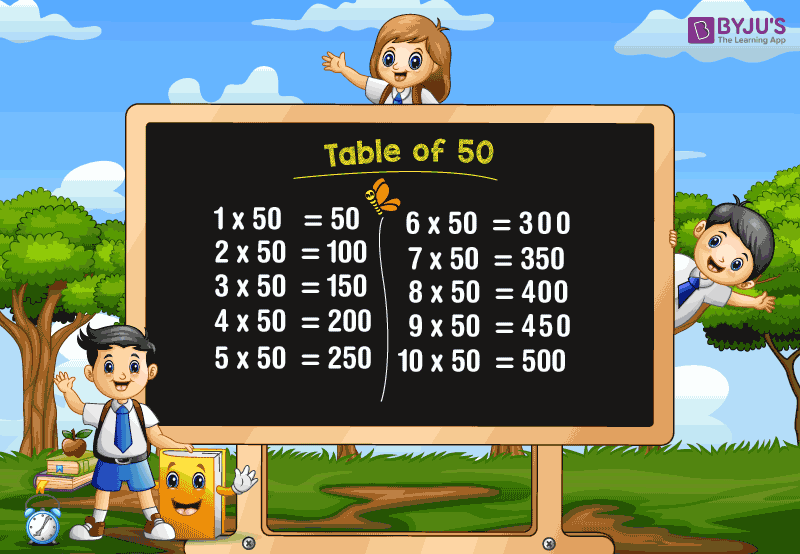# Table of 50

Table of 50 is provided here to help the students as well as the candidates who are preparing for the competitive examinations. Table of 50 is a multiplication table, which involves one of the arithmetic operations called multiplication. Students are advised to memorize the table of 50, as it saves a lot of time while writing more number of questions in a short interval of time.

### Download Table of 50 Here## 50 Times Table

A table of 50 is extremely easy if you know the table of 5. Adding “0” at the end of each answer of the 5 times tables will give the result of 50 times table.

For example, 5 times 5 is 25.

Thus, 5 times 50 is 250.

The table of 50 will result in the multiplication of the number 50 with various whole numbers.

Similarly, the repeated addition of the number “50” for the number of times also results in the 50 times table.

For example, 50 times 6 is 300.

(i.e) 50 × 6 =300.

The sum of 50 for 6 times will also give the same answer.

(i.e.) 50+50+50+50+50+50 =300

Also, check tables from 1 to 100 for more details.

## Multiplication Table of 50

Go through the following chart to learn the table of 50. The multiplication of 50 with numbers from 1 to 20 are provided below:

 50 × 1 = 50 50 × 2 = 100 50 × 3 = 150 50 × 4 = 200 50 × 5 = 250 50 × 6 = 300 50 × 7 = 350 50 × 8 = 400 50 × 9 = 450 50 × 10 = 500 50 × 11 = 550 50 × 12 = 600 50 × 13 = 650 50 × 14 = 700 50 × 15 = 750 50 × 16 = 800 50 × 17 = 850 50 × 18 = 900 50 × 19 = 950 50 × 20 = 1000

### How to Read 50 Times Table?

Read the table of 50 as follows:

• One time fifty is 50
• Two times fifty is 100
• Three times fifty is 150
• Four times fifty is 200
• Five times fifty is 250
• Six times fifty is 300
• Seven times fifty is 350
• Eight times fifty is 400
• Nine times fifty is 450
• Ten times fifty is 500
• Eleven times fifty is 550
• Twelve times fifty is 600
• Thirteen times fifty is 650
• Fourteen times fifty is 700
• Fifteen times fifty is 750.

Get More Maths Tables:

## Frequently Asked Questions on Table of 50

### 1. What is the table of 50?

The multiplication table of 50 will result in the multiples of 50. It is also called 50 times tables, which shows the multiplication of 50 with different numbers.

### 2. What is the value of 14 times 50?

14 times 50 is 700. (i.e.) 14 × 50 = 700.

### 3. What are the first 10 multiples of 50?

The first ten multiples of 50 are 50, 100, 150, 200, 250, 300, 350, 400, 450, and 500.

### 4. What is the cost of 12 books, if each book costs Rs. 50?

The cost of one book = Rs. 50
Then, the cost of 12 books = 12×50 = 600
Hence, the cost of 12 books is Rs. 600.

### 5. Find the value of x, if 50x=400.

The value of x is 8.
Explanation: 50x= 400
x = 400/50
x =8.